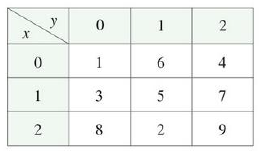Chapter 16.3, Problem 2E

Chapter
Section
Textbook Problem

A table of values of a function f with continuous gradient is given. Find  ∫C ∇ f · dr, where C has parametric equationsx = t2 + 1 y = t3 + t 0 ⩽ t ⩽ 1To determine

To find: The value of Cfdr .

Explanation

Definition:

Refer to FIGURE 1 of section 16.3 in the textbook.

Consider a smooth curve represented by a vector function r(t) , atb .

If f is the differentiable function of two or three variables with gradient vector f is continuous on curve C then Cfdr is,

Cfdr=f[r(b)]f[r(a)] (1)

Express r(t) as x and y coordinates.

r(t)=xi+yj

Substitute t2+1 for x and t3+t for y,

r(t)=(t2+1)i+(t3+t)j (2)

Find the C is smooth curve or not.

Consider r(t) is the vector function of C. If the value of differentiation of r(t) is not equal to 0 then C is a smooth curve.

Differentiate equation (2) with respect to t.

ddt[r(t)]=ddt(t2+1)i+ddt(t3+t)j

r(t)=(2t)i+(3t2+1)j

The value of t limits between 0 and 1.

The value of 3t2+10 for any values lies between 0 and 1. Therefore, C is a smooth curve

Still sussing out bartleby?

Check out a sample textbook solution.

See a sample solution

The Solution to Your Study Problems

Bartleby provides explanations to thousands of textbook problems written by our experts, many with advanced degrees!

Get Started

Change 6.4 mg to g.

Elementary Technical Mathematics

Evaluate the integrals in Problems 1-26. 25. (a) (b) (c) (d)

Mathematical Applications for the Management, Life, and Social Sciences

The average value of f(x) = 3x2 + 1 on the interval [2, 4] is: a) 29 b) 66 c) 58 d) 36

Study Guide for Stewart's Single Variable Calculus: Early Transcendentals, 8th